## A riddle wrapped in a mystery inside a balls-up

Consider #2 on the Mori-Mukai list of rank-3 Fano 3-folds.  This has been giving us some difficulty, which I have now resolved.  We were making a combination of mistakes.  Mori and Mukai describe the variety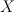$X$ as follows.

A member of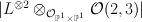$|L^{\otimes 2} \otimes_{\cO_{\PP^1 \times \PP^1}} \cO(2,3)|$ on the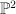$\PP^2$-bundle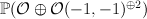$\PP(\cO \oplus \cO(-1,-1)^{\oplus 2})$ over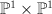$\PP^1 \times \PP^1$ such that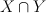$X \cap Y$ is irreducible, where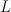$L$ is the tautological line bundle and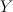$Y$ is a member of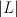$|L|$.

Our first mistake, as Mukai-sensei pointed out in an email to Corti, was using the wrong weight convention for projective bundles.  Mori and Mukai use negative weights, so the ambient$\PP^2$-bundle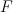$F$ is the toric variety with weight data: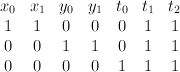$\begin{array}{cccccccc} x_0 & x_1 & y_0 & y_1 & t_0 & t_1 & t_2 & \\ 1 & 1 & 0 & 0 & 0 & 1 & 1 & \\ 0& 0 & 1 & 1 & 0 & 1 & 1 & \\ 0 & 0 & 0 & 0& 1 &1 & 1 & \end{array}$
For later convenience we change basis, expressing$F$ as the toric variety with weight data: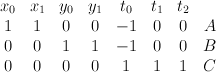$\begin{array}{cccccccc} x_0 & x_1 & y_0 & y_1 & t_0 & t_1 & t_2 & \\ 1 & 1 & 0 & 0 & -1 & 0 & 0 & A \\ 0& 0 & 1 & 1 & -1 & 0 & 0 & B \\ 0 & 0 & 0 & 0& 1 &1 & 1 & C \end{array}$$X$ is a section of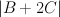$|B+2C|$.

Our second mistake was failing to accurately account for the fact that although$X$ is Fano, the bundle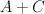$A+C$ (which restricts to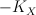$-K_X$ on$X$) is only semi-positive on$F$.  Thus we are in the situation described in this post and, in the notation defined there, we have: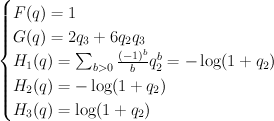$\begin{cases} F(q) = 1 \\ G(q) = 2q_3+6q_2 q_3 \\ H_1(q) = \sum_{b>0} {(-1)^{b} \over b} q_2^b = {-\log(1+q_2)} \\ H_2(q) = {-\log(1+q_2)} \\ H_3(q) = \log(1+q_2) \end{cases}$
and hence: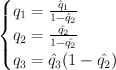$\begin{cases} q_1 = {\hat{q}_1 \over 1 - \hat{q}_2} \\ q_2 = {\hat{q_2} \over 1 - \hat{q_2}} \\ q_3 = \hat{q}_3 (1-\hat{q_2}) \end{cases}$
Thus the cohomological-degree-zero part of the J-function is: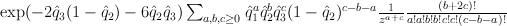$\exp({-2}\hat{q}_3(1-\hat{q}_2)-6\hat{q}_2 \hat{q}_3) \sum_{a,b,c\geq 0} \hat{q}_1^a \hat{q}_2^b \hat{q}_3^c(1-\hat{q}_2)^{c-b-a} {1 \over z^{a+c}} {(b+2c)! \over a!a!b!b!c!c!(c-b-a)!}$
and setting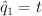$\hat{q}_1 = t$,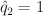$\hat{q}_2 = 1$,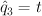$\hat{q}_3 = t$,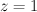$z=1$ yields: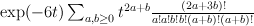$\exp(-6t) \sum_{a,b\geq 0} t^{2a+b} {(2a+3b)! \over a!a!b!b!(a+b)!(a+b)!}$
Regularizing this gives: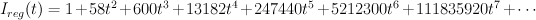$I_{reg}(t) =1+58 t^2+600 t^3+13182 t^4+247440 t^5+5212300 t^6+111835920 t^7+ \cdots$
As Galkin conjectured, this is period sequence 97.